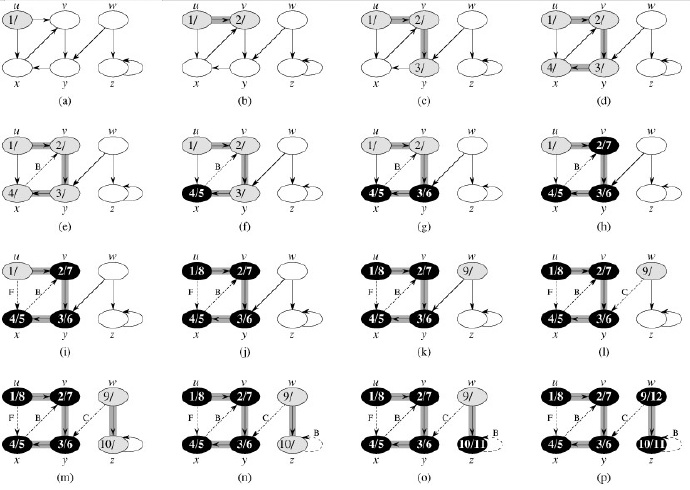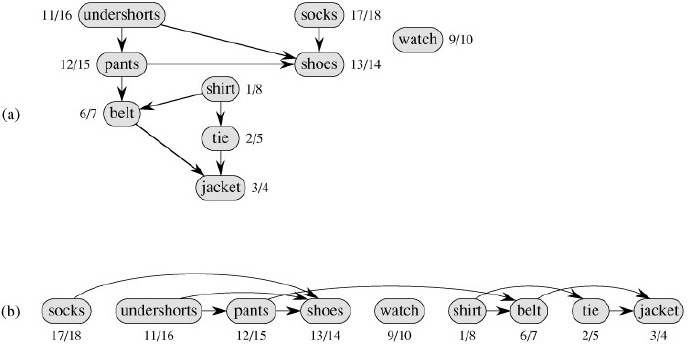# Python算法之图的遍历

更新时间：2017年11月16日 16:32:16   转载 作者：hujiawei (@五道口宅男）

Traversal就是遍历，主要是对图的遍历，也就是遍历图中的每个节点。对一个节点的遍历有两个阶段，首先是发现(discover)，然后是访问(visit)。遍历的重要性自然不必说，图中有几个算法和遍历没有关系？！

[算法导论对于发现和访问区别的非常明显，对图的算法讲解地特别好，在遍历节点的时候给节点标注它的发现节点时间d[v]和结束访问时间f[v]，然后由这些时间的一些规律得到了不少实用的定理，本节后面介绍了部分内容，感兴趣不妨阅读下算法导论原书]

Let'slookatthefollowingrelatedproblem.Showthatyoucanorderthenodesinaconnectedgraph,V1,V2,…Vn,sothatforanyi=1…n,thesubgraphoverV1,…,Viisconnected.Ifwecanshowthisandwecanfigureouthowtodotheordering,wecangothroughallthenodesinaconnectedcomponentandknowwhenthey'reallusedup.```# Walking Through a Connected Component of a Graph Represented Using Adjacency Sets
def walk(G, s, S=set()):            # Walk the graph from node s
P, Q = dict(), set()            # Predecessors + "to do" queue
P[s] = None                 # s has no predecessor
Q.add(s)                  # We plan on starting with s
while Q:                  # Still nodes to visit
u = Q.pop()               # Pick one, arbitrarily
for v in G[u].difference(P, S):     # New nodes?
Q.add(v)              # We plan to visit them!
P[v] = u              # Remember where we came from
return P```

```def some_graph():
a, b, c, d, e, f, g, h = range(8)
N = [
[b, c, d, e, f],  # a
[c, e],       # b
[d],        # c
[e],        # d
[f],        # e
[c, g, h],     # f
[f, h],       # g
[f, g]       # h
]
return N

G = some_graph()
for i in range(len(G)): G[i] = set(G[i])
print list(walk(G,0)) #[0, 1, 2, 3, 4, 5, 6, 7]```

```def components(G):               # The connected components
comp = []
seen = set()                # Nodes we've already seen
for u in G:                 # Try every starting point
if u in seen: continue         # Seen? Ignore it
C = walk(G, u)             # Traverse component
seen.update(C)             # Add keys of C to seen
comp.append(C)             # Collect the components
return comp```

```G = {
0: set([1, 2]),
1: set([0, 2]),
2: set([0, 1]),
3: set([4, 5]),
4: set([3, 5]),
5: set([3, 4])
}

print [list(sorted(C)) for C in components(G)] #[[0, 1, 2], [3, 4, 5]]```

[接下来作者作为扩展介绍了欧拉回路和哈密顿回路：前者是经过图中的所有边一次，然后回到起点；后者是经过图中的所有顶点一次，然后回到起点。网上资料甚多，感兴趣自行了解]

[注：具体的转换方式我还未明白，下面是作者给出的构造说明]

Here the “keep one hand on the wall” strategy will work nicely. One way of seeing why it works is to observe that the maze really has only one inner wall (or, to put it another way, if you put wallpaper inside it, you could use one continuous strip). Look at the outer square. As long as you're not allowed to create cycles, any obstacles you draw have to be connected to the it in exactly one place, and this doesn't create any problems for the left-hand rule. Following this traversal strategy, you'll discover all nodes and walk every passage twice (once in either direction).```def rec_dfs(G, s, S=None):
if S is None: S = set()           # Initialize the history
for u in G[s]:               # Explore neighbors
if u in S: continue           # Already visited: Skip
rec_dfs(G, u, S)            # New: Explore recursively
return S # For testing

G = some_graph()
for i in range(len(G)): G[i] = set(G[i])
print list(rec_dfs(G, 0))  #[0, 1, 2, 3, 4, 5, 6, 7]```

```def iter_dfs(G, s):
S, Q = set(), []              # Visited-set and queue
Q.append(s)                 # We plan on visiting s
while Q:                  # Planned nodes left?
u = Q.pop()               # Get one
if u in S: continue           # Already visited? Skip it
S.add(u)                # We've visited it now
Q.extend(G[u])             # Schedule all neighbors
yield u                 # Report u as visited

G = some_graph()
for i in range(len(G)): G[i] = set(G[i])
print list(iter_dfs(G, 0)) #[0, 5, 7, 6, 2, 3, 4, 1]```

```def traverse(G, s, qtype=set):
S, Q = set(), qtype()
while Q:
u = Q.pop()
if u in S: continue
for v in G[u]:
yield u```

```class stack(list):

G = some_graph()
print list(traverse(G, 0, stack)) #[0, 5, 7, 6, 2, 3, 4, 1]``````#Depth-First Search with Timestamps
def dfs(G, s, d, f, S=None, t=0):
if S is None: S = set()           # Initialize the history
d[s] = t; t += 1              # Set discover time
for u in G[s]:               # Explore neighbors
if u in S: continue           # Already visited. Skip
t = dfs(G, u, d, f, S, t)        # Recurse; update timestamp
f[s] = t; t += 1              # Set finish time
return t                  # Return timestamp```(1)白色表示该边是一条树边；

(2)灰色表示该边是一条反向边；

(3)黑色表示该边是一条正向边或者交叉边。```#Topological Sorting Based on Depth-First Search
def dfs_topsort(G):
S, res = set(), []             # History and result
def recurse(u):               # Traversal subroutine
if u in S: return            # Ignore visited nodes
for v in G[u]:
recurse(v)             # Recurse through neighbors
res.append(u)              # Finished with u: Append it
for u in G:
recurse(u)               # Cover entire graph
res.reverse()                # It's all backward so far
return res

G = {'a': set('bf'), 'b': set('cdf'), 'c': set('d'), 'd': set('ef'), 'e': set('f'), 'f': set()}
print dfs_topsort(G)```

[接下来作者介绍了一个Iterative Deepening Depth-First Search，没看懂，貌似和BFS类似]

One way of visualizing BFS and DFS is as browsing the Web. DFS is what you get if you keep following links and then use the Back button once you're done with a page. The backtracking is a bit like an “undo.” BFS is more like opening every link in a new window (or tab) behind those you already have and then closing the windows as you finish with each page.

BFS的代码很好实现，主要是使用队列

```#Breadth-First Search
from collections import deque

def bfs(G, s):
P, Q = {s: None}, deque([s])        # Parents and FIFO queue
while Q:
u = Q.popleft()             # Constant-time for deque
for v in G[u]:
if v in P: continue         # Already has parent
P[v] = u              # Reached from u: u is parent
Q.append(v)
return P

G = some_graph()
print bfs(G, 0)```

Python的list可以很好地充当stack，但是充当queue则性能很差，函数bfs中使用的是collections模块中的deque，即双端队列(double-ended queue)，它一般是使用链表来实现的，这个类有extend、append和pop等方法都是作用于队列右端的，而方法extendleft、appendleft和popleft等方法都是作用于队列左端的，它的内部实现是非常高效的。

Internally, the deque is implemented as a doubly linked list of blocks, each of which is an array of individual elements. Although asymptotically equivalent to using a linked list of individual elements, this reduces overhead and makes it more efficient in practice. For example, the expression d[k] would require traversing the first k elements of the deque d if it were a plain list. If each block contains b elements, you would only have to traverse k//b blocks.1.对原图G运行DFS，得到每个节点的完成时间f[v]；

2.得到原图的转置图GT；

3.对GT运行DFS，主循环按照节点的f[v]降序进行访问；

4.输出深度优先森林中的每棵树，也就是一个强连通分支。

```def tr(G):                   # Transpose (rev. edges of) G
GT = {}
for u in G: GT[u] = set()          # Get all the nodes in there
for u in G:
for v in G[u]:
return GT

def scc(G):
GT = tr(G)                 # Get the transposed graph
sccs, seen = [], set()
for u in dfs_topsort(G):          # DFS starting points
if u in seen: continue         # Ignore covered nodes
C = walk(GT, u, seen)          # Don't go "backward" (seen)
seen.update(C)             # We've now seen C
sccs.append(C)             # Another SCC found
return sccs

from string import ascii_lowercase
def parse_graph(s):
# print zip(ascii_lowercase, s.split("/"))
# [('a', 'bc'), ('b', 'die'), ('c', 'd'), ('d', 'ah'), ('e', 'f'), ('f', 'g'), ('g', 'eh'), ('h', 'i'), ('i', 'h')]
G = {}
for u, line in zip(ascii_lowercase, s.split("/")):
G[u] = set(line)
return G

G = parse_graph('bc/die/d/ah/f/g/eh/i/h')
print list(map(list, scc(G)))
#[['a', 'c', 'b', 'd'], ['e', 'g', 'f'], ['i', 'h']]```

[最后作者提到了一点如何进行更加高效的搜索，也就是通过分支限界来实现对搜索树的剪枝，具体使用可以看下这个问题顶点覆盖问题Vertext Cover Problem]

In Kosaraju's algorithm, we find starting nodes for the final traversal by descending finish times from an initial DFS, and we perform the traversal in the transposed graph (that is, with all edges reversed). Why couldn't we just use ascending finish times in the original graph?

Try finding a simple example where this would give the wrong answer. (You can do it with a really small graph.)

python先序遍历二叉树问题

python实现报表自动化详解

python绘制铅球的运行轨迹代码分享